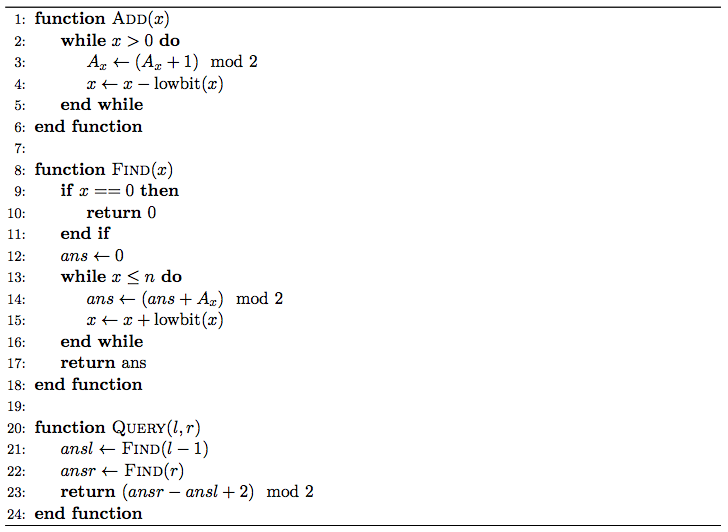# #291. 【ZJOI2017】树状数组

• $1~x$, 表示将 $A_x$ 变成 $(A_x + 1) \bmod{2}$。
• $2~l~r$, 表示询问 $(\sum_{i=l}^r A_i) \bmod{2}$。• $1~l~r$, 表示在区间 $[l, r]$ 中等概率选取一个 $x$ 并执行 $\mathrm{Add}(x)$。
• $2~l~r$, 表示询问执行 $\mathrm{Query}(l, r)$ 得到的结果是正确的概率是多少。

### 样例一

#### input

5 5
1 3 3
2 3 5
2 4 5
1 1 3
2 2 5



#### output

1
0
665496236



### 限制与约定

1$\le 5$$\le 10 2\le 50$$\le 50$
3
4$\le 3000$$\le 3000 5 6\le 10^5$$\le 10^5$所有询问都在修改后
7
8
9
10